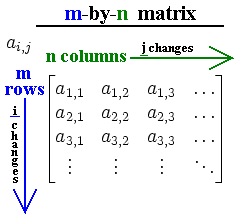# Matrix implementation and operations in C++ – Part 1

## Contents

Matrices are undoubtedly a very important topic in computer science. With the help of matrices many computational problems, ranging from finding roots of algebraic equations to extensive image manipulation and feature extraction in image processing and even video games, will become solvable. In this tutorial we will have a look into how to implement a matrix data type in c++ and also how to perform simple operations on matrices.

Please note that the c++ codes in this tutorial are mostly require to be compiled with a c++11 compatible compiler! e.g. provide ‘-std=c++11’ to g++ compiler

## Introduction

If you have any experience with one-dimensional arrays in any programming language, learning about matrices is going to be easy. A simple one-dimensional matrix, is nothing more but a rectangular array of any type (well, the most useful data types that can be related to this tutorial are integers and/or whole numbers). A good example is the following image:Graphical representation of a m-by-n matrix (courtesy of Wikipedia)

### Declaring and defining matrices in C/C++

As you can see, a matrix is just a rectangular array, meaning that each row of the matrix is just a simple one-dimensional array. In fact, the easiest way (and the dumbest way as well!) is to somehow let the computer think that one-dimensional array of the same size are forming a matrix:

The above way of coding a 3×3 matrix, while working, quickly becomes unmanageable if you want to operate on more than a few matrices with larger rows and columns. Although it could be obvious, the solution to have a more abstraction over defining matrices in C/C++ is already within the code above. What we need is a single pointer to pointer, which can represent the whole matrix:

Do not let the above code look complicated to you. All we did was obtaining a pointer, which in turn points to the first element in the matrix ai,j which will be a1,1. The other thing we did, is using the new  keyword (same as malloc() in plain C), because we expect to use the generated matrix not only in one scope of code, but perhaps we need to pass the matrix between different classes or functions, hence why we created the matrix on the heap and not the stack. Now you have to be careful about memory leakage, so you have to delete it (free the memory used by the matrix) when you are done working with the generated matrix (or matrices!):

In the function above, we get the matrix pointer as well as number of rows an columns, and we simply perform the reverse of the generateMatrix function to free up the memory space by deleting the pointers.

Now, how about a helper function which gets a matrix pointer and size of the rows and columns, and prints the content? It is nothing special, just refactoring the print section in our first code snippet, and making it compatible with our new matrix pointer, yields:

Having the necessary tools at hand, now we can perform what the first code snipped did, in a more elegant way:

We see that we now have a more clean and manageable code, but this still has some flaws. What if you need to create more matrices? then for each matrix you would need 3 variables to hold the reference to the matrix, the number of rows and the number of columns. You may already have guessed, to make this approach even more abstract we need a struct or a class to contain each matrix with all the details and helper functions which could make working with matrices easier.